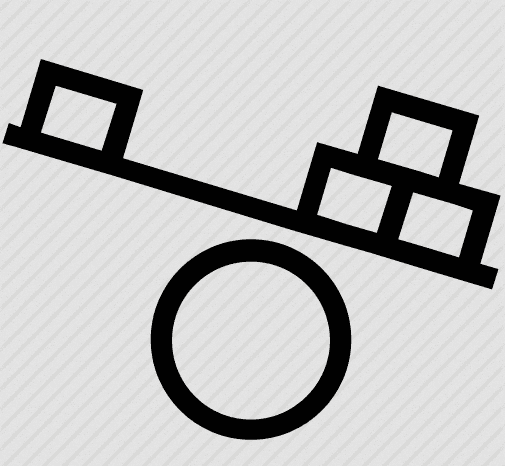# Physics Practical Investigation

• Jeff97

#### Jeff97

Homework Statement
Carry out an investigation in to the relationship between the mass of an object (m) and the distance from the fulcrum (d) at which it will cause the plank to tip.
Relevant Equations
You will use a ruler to model the plank and a pile of quarters (6) to model the mass of the cyclist
Homework Statement: Carry out an investigation into the relationship between the mass of an object (m) and the distance from the fulcrum (d) at which it will cause the plank to tip.
Homework Equations: You will use a ruler to model the plank and a pile of quarters (6) to model the mass of the cyclist

I kind of know what to do but not really. My first step is data gathering, so it says to place your ruler on a flat surface (i.e a table) then place a coin on the tipping point then add more coins and move them to find the new tipping point. My question is in my data table I need to make what are the values I need to collect? distance (d) and mass(m) the mass in the independent variable correct? and distance is the dependant? Also, how should I set up my data gathering table?

•uniquegirl

Cyclist on a teeter-totter? A diagram or picture would probably help...

I'm not given a diagram...sorry.

I'm not given a diagram...sorry.
Here you go. Happy to help...https://cdn3.iconfinder.com/data/icons/science-2-8/48/192-512.png#### Attachments

Ok, thanks. Still, I need some guidance, please.

@berkeman are they any sites that can help me than?
We are happy to help you here, but you really need to do a better job of defining the problem and showing your work.

Draw a diagram of what you are asking. Then scan it to a PDF file, or take a good quality picture of it with your cell phone to make a JPG file. Then "attach" the file to a reply here where you describe in a lot more detail what you are trying to do in this experiment.

Thank you.

What coins? What tipping point? If the ruler is flat on a table, there is no fulcrum.

Are you trying to set up a teeter-totter with coins to add weight to one side and something else on the other side?

And was the word "cycle" a typo in your first post to start this thread?

1. My first step is to set up a data gathering table, it says to place your ruler on a flat surface (i.e a table) then place a coin on the tipping point then add more coins and move them to find the new tipping point.
As the problem suggests, your first step is to gather a bunch of quarters and a ruler. Although the problem does not suggest it, you will also need a fulcrum. A small rigid metal or plastic tube, a thickish piece of wire, the back of a wooden spoon (it's stable on a flat surface), a round pencil would all be good. Then you need to decide on your independent variable. For that you need to write down the appropriate equation at the tipping point and decide what you are going to vary at will (independent variable) and what you are going to measure at the tipping point (dependent variable). Choices for these could be
1. Number of coins to the left of the fulcrum.
2. Number of coins to the right of the fulcrum.
3. Distance of fulcrum to the left side coins.
4. Distance of fulcrum to the right side coins.
5. The ratio of distances to the fulcrum.
6. The ratio of number of coins.
At this point you need to consider the theory. What does the theory predict about the expression relating all of the above quantities? You need to design an experiment such that if you vary only one of the quantities, your dependent variable will be uniquely determined. This means that you may have to keep some of the quantities fixed as you vary your independent variable. The equation relating these quantities should guide your thinking in that regard.

@berkeman Here. ... I don't know how to compare my experimental results with theory. I was thinking along the lines of of substituting a weight value and using 10cm (i.e 20cm on the table which is what D is) into d=(Mr x D)1/Mc d=(22x20)1/5.0= 8.8

So if this is right do I say in theory my balancing point for one coin should be 8.8cm off the fulcrum but I found it to be 10cm?

Note they give me d=(mrxD) 1/mq, where d= distance mr=mass ruler (22g) D is the distance that's on the other side of the fulcrum and MQ, is mass of qoauter coin (5.0g)

Last edited:
@berkman do you need me to supply you with my tables? graphs? or gradient ect ect?

Although the problem does not suggest it, you will also need a fulcrum.
Perhaps the ruler is supposed to project from the edge of the table. That would make a better defined fulcrum than would a pencil.
It leaves open which end the coins are supposed to be on.

•Jeff97
@haruspex Yes this is correct.

@berkeman Here. ... I don't know how to compare my experimental results with theory. I was thinking along the lines of substituting a weight value and using 10cm (i.e 20cm on the table which is what D is) into d=(Mr x D)1/Mc d=(22x20)1/5.0= 8.8

So if this is right do I say in theory my balancing point for one coin should be 8.8cm off the fulcrum but I found it to be 10cm?

Note they give me d=(mrxD) 1/mq, where d= distance mr=mass ruler (22g) D is the distance that's on the other side of the fulcrum and MQ, is mass of quarter coin (5.0g)
do you think you could assist me with this?